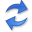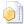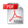Causal Inference on Event Sequences

Abstract. Given two discrete valued time series---that is, event sequences---of length $$n$$ can we tell whether they are causally related? That is, can we tell whether $$x^n$$ causes $$y^n$$, whether $$y^n$$ causes $$x^n$$? Can we do so without having to make assumptions on the distribution of these time series, or about the lag of the causal effect? And, importantly for practical application, can we do so accurately and efficiently? These are exactly the questions we answer in this paper.

We propose a causal inference framework for event sequences based on information theory. We build upon the well-known notion of Granger causality, and define causality in terms of compression. We infer that $$x^n$$ is likely a cause of $$y^n$$ if $$y^n$$ can be (much) better sequentially compressed given the past of both $$y^n$$ and $$x^n$$, than for the other way around. To compress the data we use the notion of sequential normalized maximal likelihood, which means we use minimax optimal codes with respect to a parametric family of distributions. To show this works in practice, we propose CuTe, a linear time method for inferring the causal direction between two event sequences. Empirical evaluation shows that CuTe works well in practice, is much more robust than transfer entropy, and ably reconstructs the ground truth on river flow and spike train data.

## Implementationthe Python source code (May 2018) by Kailash Budhathoki.

## Related PublicationsBudhathoki, K & Vreeken, J Causal Inference on Event Sequences. In: Proceedings of the SIAM Conference on Data Mining (SDM), pp 55-63, SIAM, 2018. (23.2% acceptance rate)